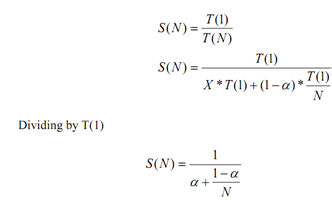## Amdahls law - calculate speed up performance, Computer Engineering

Assignment Help:

To calculate speed up performance various laws have been developed. These laws are discussed here.

Amdahl's Law

Remember, the speed up factor helps us in knowing the relative gain achieved in shifting the implementation of a task from sequential computer to parallel computer and the performance does not enhance linearly with the increase in number of processors. Due to the above reason of saturation in 1967 Amdahl's law was derived. Amdahl's law states that a program have two types of operations i.e. complete sequential operations which should be done sequentially and complete parallel operations which can be implemented on multiple processors. The statement of Amdahl's law can be described with the help of an example.

Let us take a problem say P which has to be solved using a parallel computer. According to Amdahl's law, there are generally two types of operations. Thus, the problem will have some sequential operations and some parallel operations. We already know that it needs T (1) amount of time to implement a problem using a sequential algorithm and sequential machine. The time to compute the sequential operation is a fraction α (alpha) (α ≤ 1) of the total implementation time i.e. T (1) and the time to compute the parallel operations is (1- α). Thus, S (N) can be calculated as under:#### What is indexed addressing, What is Indexed addressing The location of ...

What is Indexed addressing The location of data is calculated as sum of an address specified by one of previous methods, and value of an index register. This allows an array of

#### Pythagorean theorem, if the 6 is a and the b is 3 what is the c ?

if the 6 is a and the b is 3 what is the c ?

#### What is virtual memory, What is virtual memory? Virtual memory is a met...

What is virtual memory? Virtual memory is a method used in some large computer systems that allow the user to construct program as by a large memory space were available, equal

#### user to enter the weight, A red and blue car were involved in a head-on co...

A red and blue car were involved in a head-on collision. The red car was at a standstill and the blue car was possibly  speeding. Eye witness video recorded suddenly following the

#### Name some popular storage devices, Name some popular storage devices In...

Name some popular storage devices In contrasts with storage, which 1) retains data and programs irrespective of whether they are currently in use or not, (2) retains data

#### Determine capacity in bytes of a certain memory, A certain memory has a cap...

A certain memory has a capacity of 4K × 8 (i)  How many data input and data output lines does it have? (ii) How many address lines does it have? (iii) What is its capacity in bytes

#### Provision for data buffering, Data buffering is quite helpful for purpose o...

Data buffering is quite helpful for purpose of smoothing out gaps in speed of processor and I/O devices. Data buffers are registers that hold I/O information temporarily. I/O is pe

#### Explain parameter passing in procedures, Q. Explain Parameter Passing in Pr...

Q. Explain Parameter Passing in Procedures? Parameter passing is a very vital concept in assembly language. It makes assembly procedures more general. Parameter can be passed t

#### What is divide overflow, What is divide overflow?  The division operati...

What is divide overflow?  The division operation might result in a quotient with an overflow. Overflow happens when the length of the registers is finite and will not hold a nu